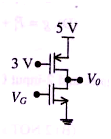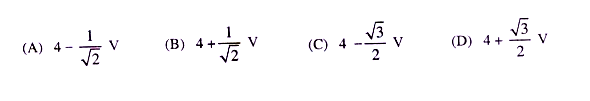## Tuesday, 4 June 2013

### Video Solutions for GATE 2009 EC : Electron Devices - Two mark questions

1. In the circuit below, the diode is ideal. The voltage V is given by

(A)   Min (Vi,1)                            (B) max (Vi,1)

(C) min(-Vi,1)                              (D) max (-Vi,1)

2. Consider the following two statements about the internal conditions in an n-channel MOSFET operating

in the active region.

S1 : The inversion charge decreases from source to drain

S2 : The channel potential increases from source to drain

Which of the following is correct ?

(A)   Both S1 and S2 is true

(B)   Both S1 and S2 is false

(C)   Both S1 and S2 is true, but S2 is not a reason for S1

(D)   Both S1 and S2 is true, and S2 is a reason for S1

Common Data Questions for 3 and 4 :

Consider a silicon P-N junction at room temperature having the following parameters :

Doping on the n-side = 1 x 1017 cm-3

Depletion width on the n-side = 0.1 um

Depletion width on the p-side = 1.0 um

Intrinsic carrier concentration = 1.4 x 1010 cm-3

Thermal voltage = 26 mV

Permittivity of free space = 8.85 x 10-14 F.cm-1

Dielectric constant of silicon = 12

3.  The built in potential of the junction is

(A)   0.70 V                    (B) 0.76 V              (C) 0.82 V

(D) cannot be estimated from the given data

4.  The peak electric field in the device is

(A)   0.15 MV.cm-1, directed from p-region to n-region

(B)   0.15 MV.cm-1, directed from n-region to p-region

(C)   1.80 MV.cm-1, directed from p-region to n-region

(D)   1.80 MV.cm-1, directed from n-region to p-region

Linked Questions : 5 and 6

Consider the CMOS circuit shown, where the gate voltage VG of the n-MOSFET is increased from zero, while the gate voltage of the p-MOSFET is kept constant at 3 volts. Assume that , for both transistors, the magnitude of the threshold voltage is 1 volt and the product of the trans conductance parameter and the (W/L) ratio, i.e. the quantity µCox.(W/L) is 1 mA. V-2.5. For small increase in VG beyond 1 Volt, which of the following fives the correct description of the region of operation of each MOSFET ?

(A)   Both the MOSFETs are in saturation region

(B)   Both the MOSFETs are in triode region

(C)   N-MOSFET is in triode region and p-MOSFET is in saturation region

(D)   N-MOSFET is in saturation region and p-MOSFET is in triode region

6.  Estimate the output voltage Vo for VG = 1.5 Volts. [Hint : Use the appropriate current voltage
equation for each MOSFET, based on the answer to above Question]